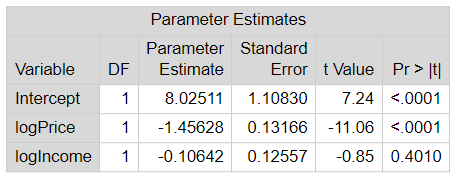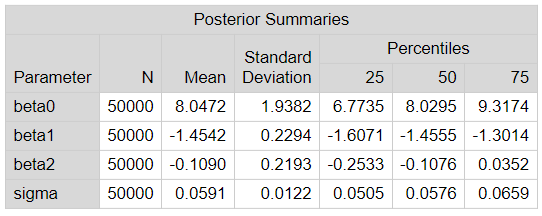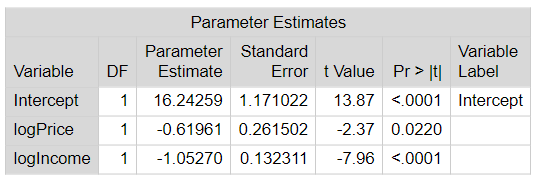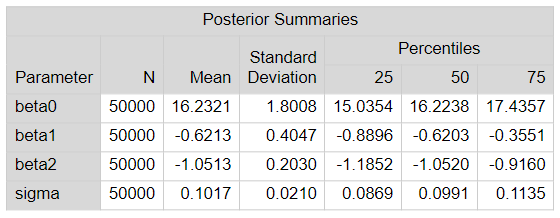## Stretching Price Elasticity Models into Bayesian

Started ‎10-17-2023 by
Modified ‎10-17-2023 by
Views 223

Elasticity models are an important econometric aspect to many researchers in marketing. Specifically, researchers are interested in how demand changes in relation to the price of a product or alternative product (cross-elasticity). In this blog, we will take two very common price elasticity model approaches and stretch them into the Bayesian environment.

Let's begin with our example. Our data focuses on cigarette sales. Our variables include: the quantity sold, the price of the product, and the average income of the region containing the store. One common analysis of price elasticity is the log-log ordinary least squares model. Before we begin our analysis, we will take the log of each variable. Taking the log allows for the interpretation of predicted parameters associated with prices to be elasticities.

With the logs of the variables taken, we construct a typical ordinary least squares regression within PROC REG.

``````proc reg data=work.model plots(unpack)=all;
output out=work.diagnostics p=logQdhat r=resid;
run; quit;``````

Looking at the results of this, we can see the estimate for the price elasticity for the cigarettes while accounting for the value of income (in the log form).Select any image to see a larger version.

Mobile users: To view the images, select the "Full" version at the bottom of the page.

This OLS model can be easily recreated within PROC MCMC.

``````proc mcmc data=work.model outpost=cigoutsimple diag=all dic propcov=quanew nbi=5000 ntu=5000 nmc=100000 thin=2
mchistory=brief plots(smooth)=all seed=27513 statistics=all monitor=(_PARMS_);

parms (beta0 beta1 beta2) 0;
parms sigma 1;

prior beta: ~ normal(0,var=1e6);
prior sigma: ~ igamma(shape=2.001,scale=1.001);

mu = beta0 + beta1 * logPrice + beta2 * logIncome;
model logQd ~ normal(mu, var=sigma);
run; quit;``````

While using the bayesian approach, you will be able to incorporate any additional (prior) information about the parameters in the model. By making adjustments to the prior statement in PROC MCMC, you can deviate from the non-informative prior that we used here. Since we used a non-informative prior for all parameters, our posterior means will emulate the estimates from the classical approach.In this case, there is an issue with the regression model. Looking at the residual plot for logPrice, we see that there is a pattern (curvilinear) visible. Besides this being an issue with the assumptions of an OLS model, this is also, in our example, referred to as endogeneity. Our interpretation and use of the results are now in question.One method of dealing with endogenaity is to run a two-stage OLS model. The first stage is to take the problematic or endogenous input variable (logprice) and regress it against other variable(s) (instuments) that are correlated with logprice. Using this first stage OLS, we create predicted values of logprice and use these predictions as the input to the second stage OLS that will return us to our original model that we tried earlier. This can be performed using PROC SYSLIN.  For further details on endogeneity, check out Greene's book (Greene,William H. (1993). Econometric Analysis (3rd. ed.) Prentice-Hall.).

``````proc syslin data = work.diagnostics 2SLS out=syslin_output plots(only unpack)=(QQ RESIDHISTOGRAM);
endogenous logPrice;
instruments sales_tax;
output r=residuals;
run; quit;``````

Here are the results of this two-stage OLS. The estimated own price elasticity estimate has changed because of mitigating the endogeneity.How is this implemented within a Bayesian approach? We will run PROC MCMC twice and incorporate the use of the PREDDIST statement.

First let's run the first-stage OLS. The PREDDIST statement is taking the original training data and creating posterior predictive distributions for each observation (logprice). We save the posterior summary statistics into a new SAS data set.

``````proc mcmc data=work.model outpost=cigout2stage diag=all dic propcov=quanew nbi=5000 ntu=5000 nmc=100000 thin=2
mchistory=brief plots(smooth)=all seed=27513 statistics=all monitor=(_PARMS_);

parms (alpha0 alpha1) 0;
parms (sigma2) 1;

prior alpha: ~ normal(0,var=1e6);
prior sigma: ~ igamma(shape=2.001,scale=1.001);

mu = alpha0 + alpha1 * sales_tax;
model logPrice ~ normal(mu, var=sigma2);
preddist outpred=pred stats=summary;
ods output predsummaries=prediction_summaries;
run; quit;``````

The original data and the posterior summary statistics data sets match up nicely by observation. Using a simple merge, we combine these two data sets.

``````data newmodel;
merge work.model work.prediction_summaries;
run; quit;``````

From the posterior summary statisics, we have the choice to use the posterior mean or posterior median as our point estimate for logprice in the second-stage OLS. In our example, we use the posterior mean.

``````proc mcmc data=work.newmodel outpost=cigout2stagealt diag=all dic propcov=quanew nbi=5000 ntu=5000 nmc=100000 thin=2
mchistory=brief plots(smooth)=all seed=27513 statistics=all monitor=(_PARMS_);

parms (beta0 beta1 beta2) 0;
parms (sigma) 1;

prior beta: ~ normal(0,var=1e6);
prior sigma: ~ igamma(shape=2.001,scale=1.001);

mu = beta0 + beta1 * mean + beta2 * logIncome;
model logQd ~ normal(mu, var=sigma);
run; quit;``````

Again, we are using non-informative priors for this example. If we did have additional information about the parameters, we could have made the appropriate adjustments to the PRIOR statements in the code. Since we are using non-informative priors, our posterior means for the second-stage OLS are comparable to the solution from PROC SYSLIN.Using PROC MCMC allows one to use the powerful Bayesian techniques in elasticity models. This can lead to better understanding of the price elasticities since we now can incorporate prior information and end with posterior distributions of the parameters. If you would like more information about price elasticity models, here is a suggested article to get you started.

Find more articles from SAS Global Enablement and Learning here.

Version history
Last update:
‎10-17-2023 10:53 AM
Updated by:Contributors
Article Labels
Article Tags